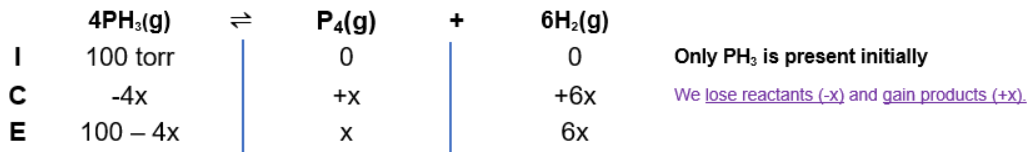# Problem: Phosphine, PH3(g), decomposes according to the equation4PH3(g) →P4(g) + 6H2(g) Time (min)        Ptotal (Torr) 0.00                  10040.0                   15180.0                   168100                     171The kinetics of the decomposition of phosphine at 950 K was followed by measuring the total pressure in the system as a function of time. The data to the right were obtained in a run where the reaction chamber contained only pure phosphine at the start of the reaction. Choose the rate law that describes this reaction.a) rate = k(PPH3)2 b) rate = k(PPH3)-2c) rate = kd) rate = k(PPH3)e) rate = k(PPH3)-1Calculate the value of the rate constant and choose the correct units

###### FREE Expert Solution

To choose the rate law that describes this reaction.

4PH3(g) →P4(g) + 6H2(g)

Step 1: ICE Chart

at t = 0, Ptot  = PPH3Step 2: Solve for x for t = 40.0 and t = 80.0 (for comparison)

Based on Dalton’s Law, the total pressure is the sum of the partial pressure of the individual gases.

t = 40.0 mins:

Ptot = PPH3 + PP4 + PH2

151 = 100 - 4x + x + 6x

3x = 151 - 100

x = 17 torr

after 40 mins:

PPH3  = 100 - 4(17)

PPH= 32 torr

t = 80.0 mins:

Ptot = PPH3 + PP4 + PH2

168 = 100 - 4x + x + 6x

3x = 168 - 100

x = 22.67 torr

after 80 mins:

PPH3  = 100 - 4(17)

PPH= 9.33 torr

Step 3: Check if 1st order reaction: rate = k[PH3]

• exponent of reactant = order of reaction (if 1, not written)
• kt=40 = kt=80

integrated rate law for a first-order reaction:

$\overline{){\mathbf{ln}}{{\mathbf{P}}}_{{\mathbf{t}}}{\mathbf{=}}{\mathbf{-}}{\mathbf{kt}}{\mathbf{+}}{{\mathbf{lnP}}}_{{\mathbf{0}}}}$

Solve for k at t = 40.0 mins:

89% (381 ratings)###### Problem Details

Phosphine, PH3(g), decomposes according to the equation

4PH3(g) →P4(g) + 6H2(g)

Time (min)        Ptotal (Torr)

0.00                  100

40.0                   151

80.0                   168

100                     171

The kinetics of the decomposition of phosphine at 950 K was followed by measuring the total pressure in the system as a function of time. The data to the right were obtained in a run where the reaction chamber contained only pure phosphine at the start of the reaction.

Choose the rate law that describes this reaction.

a) rate = k(PPH3)2

b) rate = k(PPH3)-2

c) rate = k

d) rate = k(PPH3)

e) rate = k(PPH3)-1

Calculate the value of the rate constant and choose the correct units